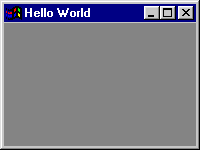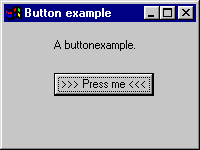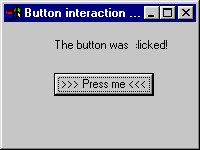# wxPerl: Another GUI for Perl### wxPerl?

If you don’t just use Perl for creating CGI scripts, you’ll probably have to create some kind of front-end for your applications sooner or later. You might use the Curses library, but if you want a nice GUI, you will probably use Tk. It’s certainly the most stable, best documented and widest used GUI that’s available for Perl. However, more and more people are using other GUIs, such as Gtk and Win32::GUI. The main reason for this is probably that Tk does not have the slickest interface that exactly matches the environment that people use. Tk has a motif-like interface, while Gnome users will want the Gtk look-and-feel, and Windows users will want the Windows look-and-feel. Of course, Tk looks more like Windows when you use it on a Win32 machine and looks more like Gtk when you run it under Gnome, but still it is a different interface.

Recently, I discovered another GUI for Perl. No, not the unmaintained Qt and FWTK modules, but wxPerl, which is being developed by Mattia Barbon. wxPerl is the Perl binding for wxWindows (http://www.wxwindows.org), which is a cross-platform GUI library for C++. When I say cross-platform, I indeed mean cross-platform: There is wxWindows for Windows, Gtk, Motif and Macintosh. wxWindows has been developed since 1992 with version 2 (the current version) being developed since 1997. It’s not a GUI that has been ported from a certain platform where it had its roots: wx stands for Windows and X – it has been designed to be cross-platform. Also it has been around for a while so it has had the chance to become a stable product.

### The wxPerl approach

wxPerl has a very rich set of standard widgets (called ``controls” in Wx-terms), ranging from simple buttons to complex HTML windows and Font dialogs. This makes it a good GUI to create full-featured applications. All needed controls are available ``off the shelf,” and if there is still a complex control you want to create yourself, then you can do so with little effort. This is a particularly big difference from, for example, Tk, where it is a real pain to define new widgets.

Programming wxPerl is different. It’s not better or worse than the Tk or Gtk interfaces, but it’s a totally different approach. The main reason for this is the source of the library, which is a C++ library. It is less Perlish - but it’s more OO.

When you want to create a new wxPerl application you start creating a new class that inherits from `Wx::App`. This subclass has to have at least one method called `OnInit` which defines the windows (called ``Frames” in Wx-terms) the application uses. If you want a default window, you use the default classes. If you want to add controls to a window, you subclass a default windowclass and add the controls to it.

This is a much more object-oriented approach than Tk and Gtk use. But unfortunately it lacks the named parameter approach Tk uses, which makes Tk look more Perlish.

Currently, there is one big disadvantage to wxPerl: It is very poorly documented. That is to say: wxWindows has lots of documentation. And if you try hard enough, then you can use that documentation for wxPerl. That takes quite a bit of effort, though. But after reading this first wxPerl tutorial you might become interested and find your own way into wxPerl.

### Hello World!

Like every tutorial, this tutorial has its own ``Hello World!” application to get started and create the first application. Examine this script:

``````   =1= #!/usr/bin/perl -w
=2= use strict;
=3= use Wx;
=4=
=5= ###########################################################
=6= #
=7= # Define our HelloWorld class that extends Wx::App
=8= #
=9= package HelloWorld;
=10=
=11= use base qw(Wx::App);   # Inherit from Wx::App
=12=
=13= sub OnInit
=14= # Every application has its own OnInit method that will
=15= # be called when the constructor is called.
=16= {
=17=    my \$self = shift;
=18=    my \$frame = Wx::Frame->new( undef,         # Parent window
=19=                                -1,            # Window id
=20=                                'Hello World', # Title
=21=                                [1,1],         # position X, Y
=22=                                [200, 150]     # size X, Y
=23=                              );
=24=   \$self->SetTopWindow(\$frame);    # Define the toplevel window
=25=   \$frame->Show(1);                # Show the frame
=26= }
=27=
=28= ###########################################################
=29= #
=30= # The main program
=31= #
=32= package main;
=33=
=34= my \$wxobj = HelloWorld->new(); # New HelloWorld application
=35= \$wxobj->MainLoop;
``````

Like every well-written Perl application, this one also begins with `-w` and `use strict`. After that we `use Wx`, the main wxPerl module. On line 9 we start our package HelloWorld, which inherits (line 11) from `Wx::App`, like all wxPerl applications. This new application now needs to have an `OnInit` method that defines the Frames (line 18) and defines which of the Frames is the TopWindow (line 24). Finally, we call the `Show` method (line 25), which makes the created `\$frame` visible.

The frame is created using a few parameters. The first is the parent window. If we were creating two frames, then the second one could be appointed as a child of the first by using `\$frame` as the first parameter of the constructor of the second frame. But in our example we have only one window, so the parent window is `undef`.

At this moment we don’t care about the second parameter (the window id, -1 means the default value), but the third and fourth are more interesting. They define the position on the screen and the size respectively. These parameters are passed as array references, and could also be the predefined `wxDefaultPosition` and `wxDefaultSize` respectively.

After defining the HelloWorld package, we have to create the main program by defining the main package (line 32). This package creates a new Wx object (line 34) out of our defined HelloWorld package and then calls the `MainLoop` method on it (line 35).

The MainLoop is the only thing that resembles to the Tk and Gtk GUIs. The whole approach of defining a new subclass of `Wx::App` is totally different.

When you execute this first example, it will look like this:### Fill the empty window.

So this was a simple example that creates an empty window with a title named ``Hello World!” Not really exciting, huh? Now we want to see more controls in the window. Let’s see how we can add a useless button that does nothing and a piece of text on the screen.

We need to have a bit of background information on how wxPerl applications (and wxWindows applications) work in general, before we can create something inside the window. As you saw in the previous example, to create an application, we need to subclass `Wx::App`. To create our own contents in a frame, we first need to subclass `Wx::Frame` and create an instance of that subclassed frame in the OnInit method of the newly created subclass of `Wx::App`.

To put controls in our subclassed frame, you first have to create a Panel inside that frame, since controls can only be placed on an instance of `Wx::Panel`. To be able to access and modify properties of the Panel and other things that you want to put inside a Frame, you will have make those items objects of the Frame.

That’s a lot of (potentially) confusing information. Let’s take this example:

``````   =1= #!/usr/bin/perl -w
=2= use strict;
=3= use Wx;
=4=
=5= ###########################################################
=6= #
=7= # Extend the Frame class to our needs
=8= #
=9= package MyFrame;
=10=
=11= use base qw(Wx::Frame); # Inherit from Wx::Frame
=12=
=13= sub new
=14= {
=15=     my \$class = shift;
=16=     my \$self = \$class->SUPER::new(@_); # call the superclass' constructor
=17=
=18=     # Then define a Panel to put the button on
=19=     my \$panel = Wx::Panel->new( \$self,  # parent
=20=                                 -1      # id
=21=                               );
=22=     \$self->{txt} = Wx::StaticText->new( \$panel,             # parent
=23=                                         1,                  # id
=24=                                         "A buttonexample.", # label
=25=                                         [50, 15]            # position
=26=                                        );
=27=     \$self->{btn} = Wx::Button->new(     \$panel,             # parent
=28=                                         1,                  # id
=29=                                         ">>> Press me <<<", # label
=30=                                         [50,50]             # position
=31=                                        );
=32=     return \$self;
=33= }
=34=
=35= ###########################################################
=36= #
=37= # Define our ButtonApp class that extends Wx::App
=38= #
=39= package ButtonApp;
=40=
=41= use base qw(Wx::App);   # Inherit from Wx::App
=42=
=43= sub OnInit
=44= {
=45=     my \$self = shift;
=46=     my \$frame = MyFrame->new(    undef,         # Parent window
=47=                                  -1,            # Window id
=48=                                  'Button example', # Title
=49=                                  [1,1],         # position X, Y
=50=                                  [200, 150]     # size X, Y
=51=                                );
=52=     \$self->SetTopWindow(\$frame);    # Define the toplevel window
=53=     \$frame->Show(1);                # Show the frame
=54= }
=55=
=56= ###########################################################
=57= #
=58= # The main program
=59= #
=60= package main;
=61=
=62= my \$wxobj = ButtonApp->new(); # New ButtonApp application
=63= \$wxobj->MainLoop;
``````

You can see here that again we define a subclass of `Wx::App` called ButtonApp (line 39). Only this time the created frame is not a `Wx::Frame` instance, but a `MyFrame` instance. This `MyFrame` is a new subclass of `Wx::Frame` that we define in line 9.

Basically we only have to override the `new` constructor of `Wx::Frame`. We want to extend the `Wx::Frame` class, so our constructor first calls its `SUPER`class’ constructor, and defines its extensions after that. Our extensions consist of a new `Panel` (line 19), which has a `StaticText` (line 22) and a `Button` (line 27) on it. Just like the original `Wx::Frame` class would do, our constructor also returns `\$self` (line 32), which finishes the definition of `MyFrame`.

As you can see, we’ve defined the `Button` and the `StaticText` objects as attributes of `MyFrame`. This is not strictly neccesary now, but if we want to add some interaction to this script, which we will do in the next example, we want to access those objects. Since they’re now stored as attributes of `MyFrame` we can access the `Button` and `StaticText` everywhere we have access to the `MyFrame` object. So it’s just a matter of style that it’s stored this way here, because we don’t actually do anything with it in this example.

When you execute this example it will look like this:But what does it do? Err … it does nothing - yet. But a GUI application without interaction is useless. So we’re going to implement some interaction. I already explained in the previous example: If you want to change the properties of the defined objects, then you will have to define them as attributes of the Frame object. That way you can always access any attribute of the object, be it a StaticText, a Button or a Menu.

Consider the following code:

``````   =1= #!/usr/bin/perl -w
=2= use strict;
=3= use Wx;
=4=
=5= ###########################################################
=6= #
=7= # Extend the Frame class to our needs
=8= #
=9= package MyFrame;
=10=
=11= use Wx::Event qw( EVT_BUTTON );
=12=
=13= use base qw/Wx::Frame/; # Inherit from Wx::Frame
=14=
=15= sub new
=16= {
=17=  my \$class = shift;
=18=
=19=  my \$self = \$class->SUPER::new(@_);  # call the superclass' constructor
=20=
=21=     # Then define a Panel to put the button on
=22=  my \$panel = Wx::Panel->new( \$self,  # parent
=23=                              -1      # id
=24=                            );
=25=
=26=  \$self->{txt} = Wx::StaticText->new( \$panel,             # parent
=27=                                      1,                  # id
=28=                                      "A buttonexample.", # label
=29=                                      [50, 15]            # position
=30=                                     );
=31=
=32=  my \$BTNID = 1;  # store the id of the button in \$BTNID
=33=
=34=  \$self->{btn} = Wx::Button->new(     \$panel,             # parent
=35=                                      \$BTNID,             # ButtonID
=36=                                      ">>> Press me <<<", # label
=37=                                      [50,50]             # position
=38=                                     );
=39=
=40=  EVT_BUTTON( \$self,          # Object to bind to
=41=              \$BTNID,         # ButtonID
=42=              \&ButtonClicked # Subroutine to execute
=43=             );
=44=
=45=  return \$self;
=46= }
=47=
=48= sub ButtonClicked
=49= {
=50=  my( \$self, \$event ) = @_;
=51=  # Change the contents of \$self->{txt}
=52=  \$self->{txt}->SetLabel("The button was clicked!");
=53= }
=54=
=55= ###########################################################
=56= #
=57= # Define our ButtonApp2 class that extends Wx::App
=58= #
=59= package ButtonApp2;
=60=
=61= use base qw(Wx::App);   # Inherit from Wx::App
=62=
=63= sub OnInit
=64= {
=65=     my \$self = shift;
=66=     my \$frame = MyFrame->new(   undef,         # Parent window
=67=                                 -1,            # Window id
=68=                                 'Button interaction example', # Title
=69=                                 [1,1],         # position X, Y
=70=                                 [200, 150]     # size X, Y
=71=                                );
=72=     \$self->SetTopWindow(\$frame);    # Define the toplevel window
=73=     \$frame->Show(1);                # Show the frame
=74= }
=75=
=76= ###########################################################
=77= #
=78= # The main program
=79= #
=80= package main;
=81=
=82= my \$wxobj = ButtonApp2->new(); # New ButtonApp application
=83= \$wxobj->MainLoop;
``````

This example is basically the same as the previous one, but the main difference here is the addition of some interaction. In the previous example, nothing happened when you tried to click the button. This time clicking the button will alter the text of the `StaticText` object. Let’s see what has been changed in the code:

First of all, we use `Wx::Event` and import `EVT_BUTTON`. `EVT_BUTTON` is the event handling subroutine for button-events. There are many more event handlers available, but we only need this one now.

On line 31 I’m introducing a variable to hold the button id called `\$BTNID`. I could still have used the hard-coded `1` I used in the previous example, but by using this variable it will be clearer to see where I’m referring to it. For example, it’s needed for the `EVT_BUTTON` we call at line 40. This is where we define what to do when the button is clicked. It takes the `\$self` object, the `\$BTNID` and a subroutine reference as parameters. On line 48 we define that subroutine.

An event callback in wxPerl always takes two parameters: the first is the object to which it belongs (which caused the event to happen) and the second is the event object itself. In our case we don’t need that second parameter, but we do need the first, because we want to change the text of the `StaticText` object. This is the place where we see the use of defining the `StaticText` object as attribute of the `MyFrame` object. We can now simply call the `SetLabel` method on that attribute (line 52).

Before we press the button, the window will look like the one in the previous example. After we press the button, the application window will look like this:### Conclusion

I’ve shown a bit of the way wxPerl works. More precisely, I’ve shown how you can work with wxPerl. It’s obvious that this is a different approach from other GUIs. I admit that at first I myself thought this was an unnatural way of programming Perl, not to mention programming Perl GUIs. But having done some exercises, I get the feeling this is in fact a more natural approach than Tk or Gtk use. Of course, it all comes down to a matter of taste. And there’s no accounting for taste.

In the next wxPerl tutorial, I will show you how to create menus, show some more event handling and I’ll even add some more advanced controls. But the goal will be the same: to show you the hidden beauties of wxPerl!

Tags

### Feedback

Something wrong with this article? Help us out by opening an issue or pull request on GitHub